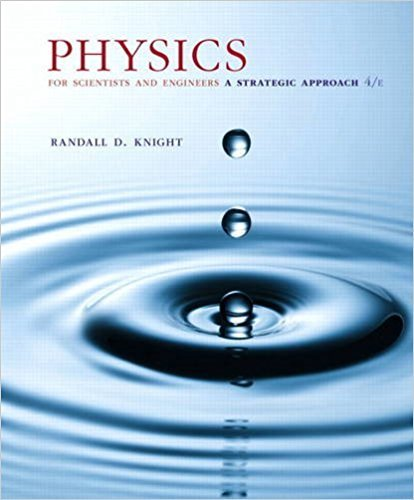×
×ISBN: 9780134081496 191

## Solution for problem 41.63 Chapter 41

Physics for Scientists and Engineers: A Strategic Approach, Standard Edition (Chs 1-36) | 4th Edition

• Textbook Solutions
• 2901 Step-by-step solutions solved by professors and subject experts
• Get 24/7 help from StudySoup virtual teaching assistantsPhysics for Scientists and Engineers: A Strategic Approach, Standard Edition (Chs 1-36) | 4th Edition

4 5 0 369 Reviews
10
2
Problem 41.63

Calculate (a) the radial wave function and (b) the radial probability density at r = 1 2 aB for an electron in the 1s state of hydrogen. Give your answers in terms of aB.

Step-by-Step Solution:
Step 1 of 3
Step 2 of 3

Step 3 of 3

##### ISBN: 9780134081496

Since the solution to 41.63 from 41 chapter was answered, more than 227 students have viewed the full step-by-step answer. Physics for Scientists and Engineers: A Strategic Approach, Standard Edition (Chs 1-36) was written by and is associated to the ISBN: 9780134081496. The full step-by-step solution to problem: 41.63 from chapter: 41 was answered by , our top Physics solution expert on 12/28/17, 08:06PM. This textbook survival guide was created for the textbook: Physics for Scientists and Engineers: A Strategic Approach, Standard Edition (Chs 1-36), edition: 4. This full solution covers the following key subjects: . This expansive textbook survival guide covers 42 chapters, and 4463 solutions. The answer to “Calculate (a) the radial wave function and (b) the radial probability density at r = 1 2 aB for an electron in the 1s state of hydrogen. Give your answers in terms of aB.” is broken down into a number of easy to follow steps, and 34 words.

Unlock Textbook Solution